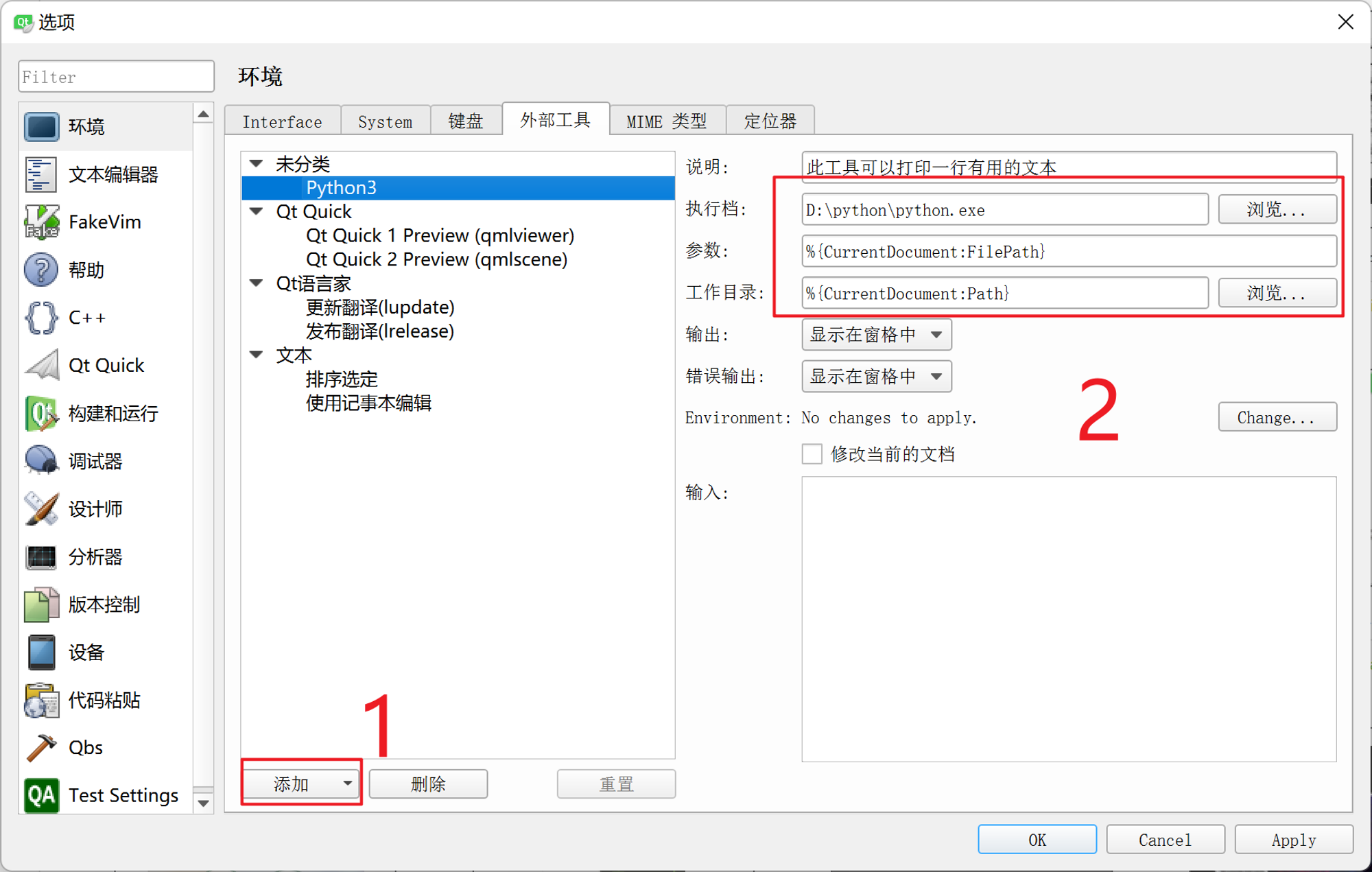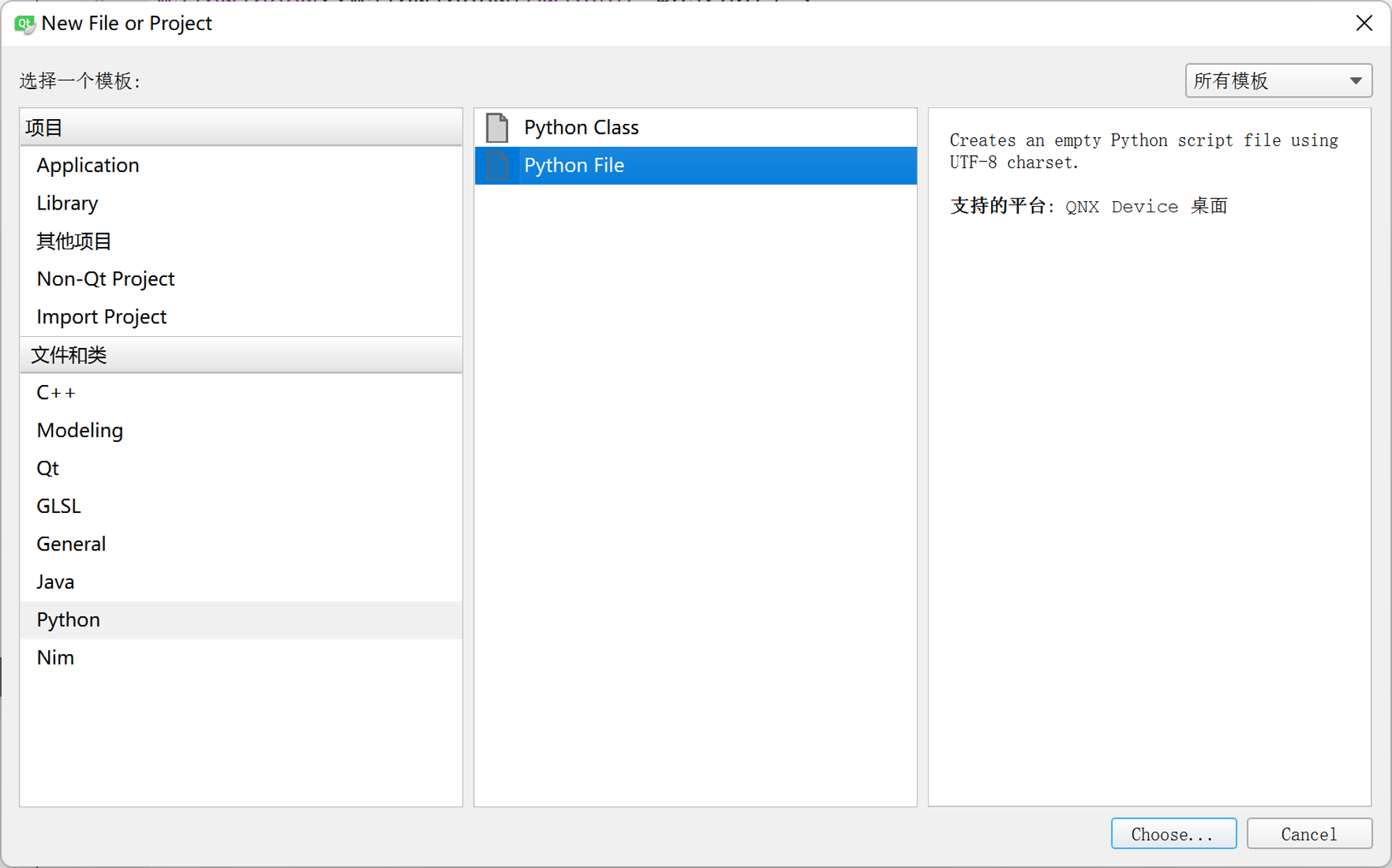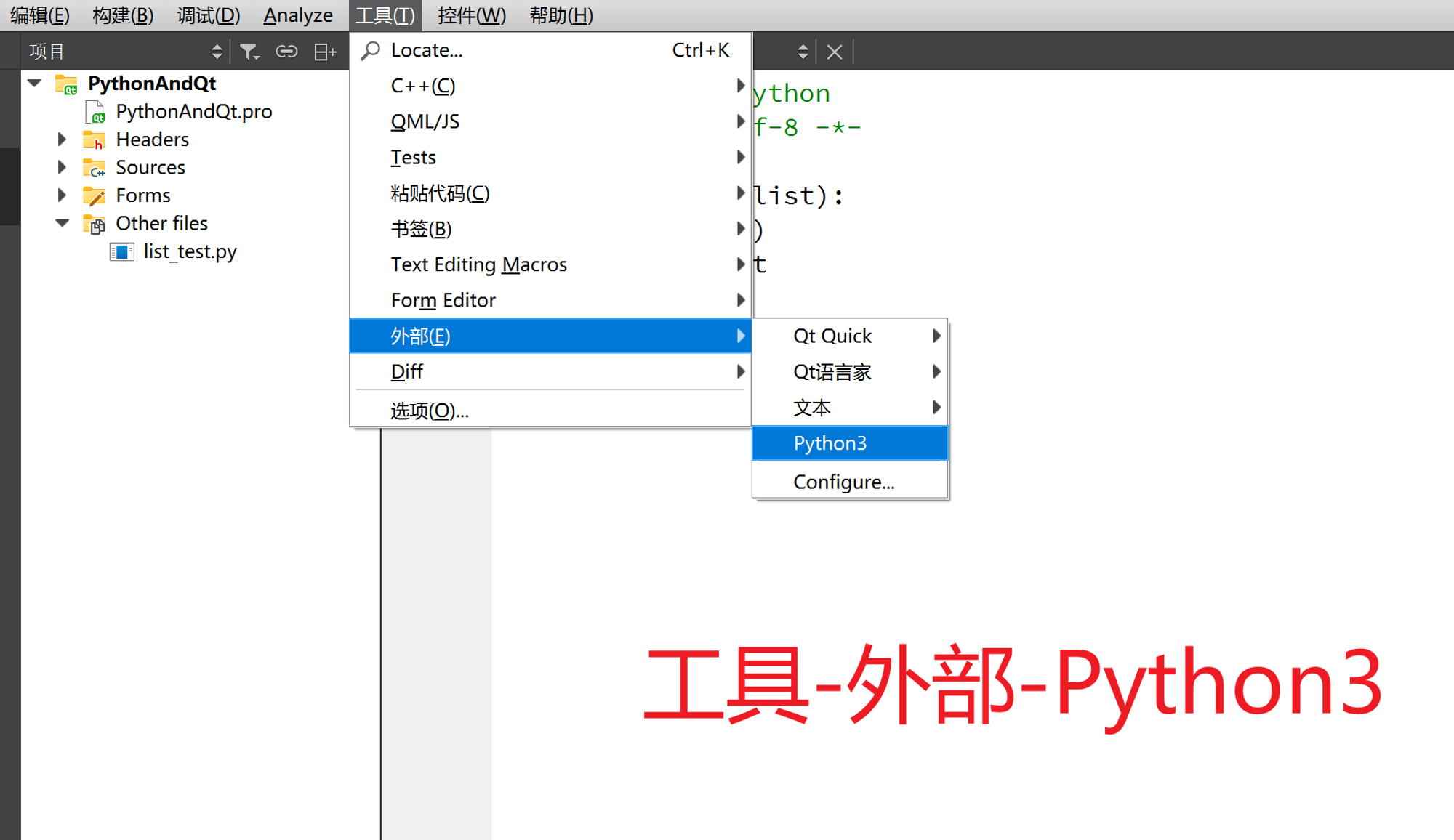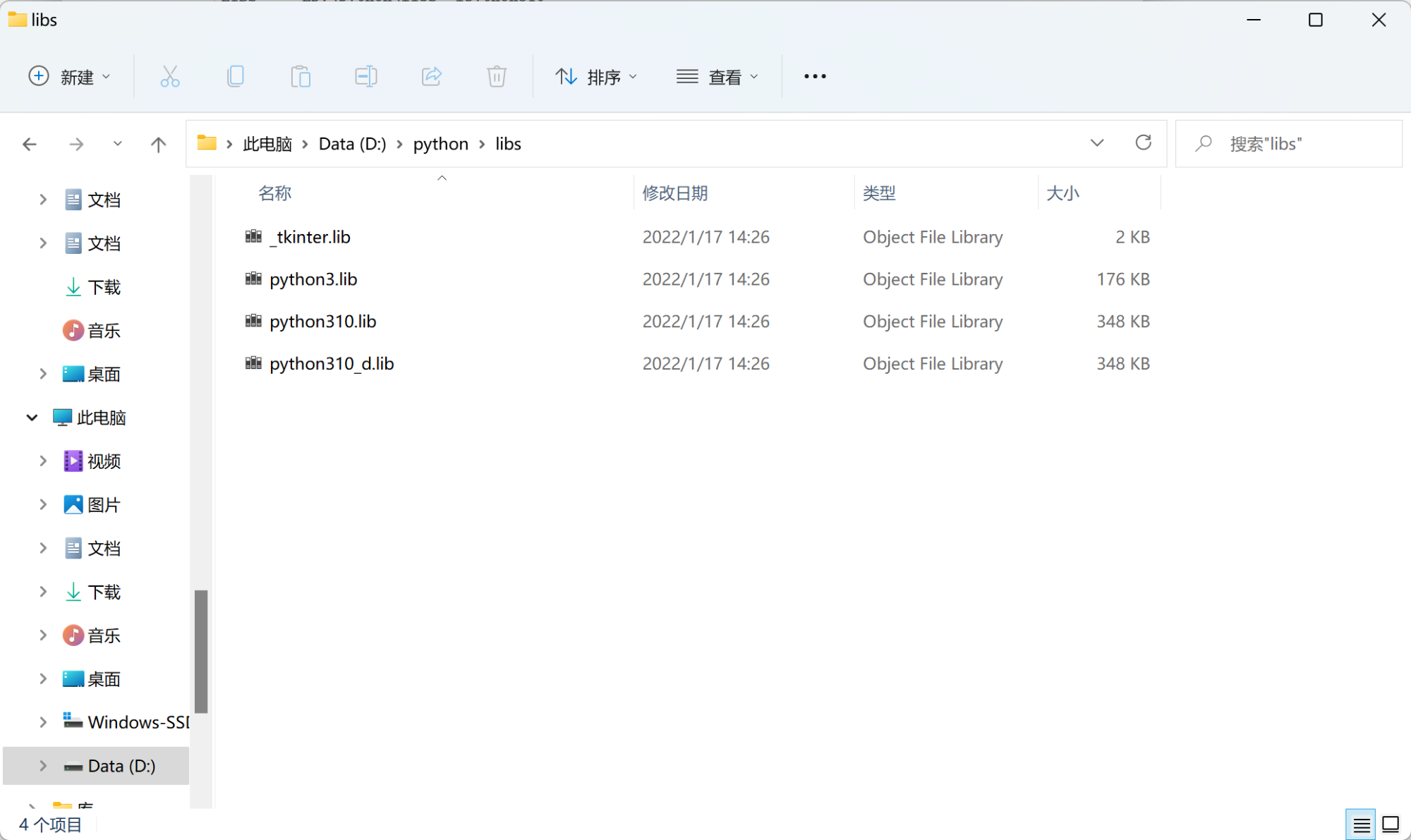K

Kern

V1

2023/03/09阅读：49主题：橙心

# Qt C++ Python 混合编程测试文档

## 环境版本

• Qt：5.9.0 （MSVC 2017 64bit）
• Python： 3.10.2 (64 bit)

## 开发步骤

### 将 Python 集成到 Qt 中

#### Qt 配置 Python 解释器#### 添加 Python 脚本文件``# list_test.pyimport numpydef sort_by_list(mylist):    print('使用python列表')    mylist.sort()    return mylistdef sort_by_numpy_list(myarray):    # if type(myarray) is not numpy.ndarray:    #     return    print('使用numpy数组')    myarray.sort()    return myarray``### Qt 调用Python脚本

#### pro 导入Python环境

``INCLUDEPATH += -I D:\python\includeLIBS += -LD:\python\libs -lpython310``

• `+= -LD:\python` 中的 LD 不可分开
• `-lpython310` 根据自己python版本决定，比如python 3.9 为 `-lpython39`
• 我的python路径如下所示：### C++ 调用 Python 函数接口 API介绍

• 初始化Python解释器
• 从C++到Python转换数据
• 用转换后的数据做参数调用Python函数
• 把函数返回值转换为C++数据结构

#### 初始化Python解释器

``#include <Python.h>...Py_Initialize();PyRun_SimpleString("import sys");PyRun_SimpleString("sys.argv = ['python.py']");PyRun_SimpleString("sys.path.append('./')");``

#### C++ 数据转化为 PyObject

PyObject 为 C++ 中 Python 的数据类型，传入 Python 函数的参数和从 Python 函数得到的返回值，必须以 PyObject 的形式存在。

• 可以采用下面的库函数进行基本数据的转换：
``PyObject* PyLong_FromLong(long v)PyObject* PyBool_FromLong(long v)PyObject* PyFloat_FromDouble(double v)``
• 可以采用 Py_BuildValue() 函数进行转换

`PyObject *Py_BuildValue(const char **format*, ...)`

``Py_BuildValue("")  None Py_BuildValue("i", 123)  123 Py_BuildValue("iii", 123, 456, 789)  (123, 456, 789) Py_BuildValue("s", "hello")  'hello' Py_BuildValue("ss", "hello", "world")  ('hello', 'world') Py_BuildValue("s#", "hello", 4) 'hell' Py_BuildValue("()")  () Py_BuildValue("(i)", 123)  (123,) Py_BuildValue("(ii)", 123, 456) (123, 456) Py_BuildValue("(i,i)", 123, 456) (123, 456) Py_BuildValue("[i,i]", 123, 456) [123, 456]  Py_BuildValue("{s:i,s:i}", "abc", 123, "def", 456) {'abc': 123, 'def': 456} Py_BuildValue("((ii)(ii)) (ii)", 1, 2, 3, 4, 5, 6) (((1, 2), (3, 4)), (5, 6))``
• 列表、元组类型转换 PyList、PyTuple

List API 简单介绍

``// 判断是否是一个Python List（列表）int PyList_Check(PyObject *p) // 创建一个列表PyObject* PyList_New(Py_ssize_t len) // 获取列表元素的个数 len(list)Py_ssize_t PyList_Size(PyObject *list) // 和PyList_Size 一样，但是就是没有错误检查Py_ssize_t PyList_GET_SIZE(PyObject *list) // 从列表里面获取一个元素,计数器不会加1PyObject PyList_GetItem(PyObject list, Py_ssize_t index) // 和PyList_GetItem一样，但是就是没有错误检查PyObject PyList_GET_ITEM(PyObject list, Py_ssize_t i) // 设置别表指定位置的值，下标的所在的位置必须是有值的，并且是有效的int PyList_SetItem(PyObject list, Py_ssize_t index, PyObject item) // 和PyList_SetItem一样，但是就是没有错误检查void PyList_SET_ITEM(PyObject list, Py_ssize_t i, PyObject o) // 在列表指定位置插入值 list.insert(index, item)int PyList_Insert(PyObject list, Py_ssize_t index, PyObject item) // 在列表尾部追加值 list.append(item)int PyList_Append(PyObject list, PyObject item) // 获取列表里面一段切片数据，一段指定范围的数据 list[low:higt]PyObject PyList_GetSlice(PyObject list, Py_ssize_t low, Py_ssize_t high) // 设置列表分片数据，指定列表范围的数据 list[low:higt] = itemlistint PyList_SetSlice(PyObject list, Py_ssize_t low, Py_ssize_t high, PyObject itemlist) // 对列表数据进行排序 list.sort()int PyList_Sort(PyObject *list) // 把列表里面的所有数据反转 list.reverse()int PyList_Reverse(PyObject *list) // 将Python列表转为Python元组 tuple(list)PyObject PyList_AsTuple(PyObject list) ``

``// 将C++ 数组 array_1 转化为 PyList PyObject *PyList = PyList_New(SizeOfList) // 定义一个长度为 SizeOfList 的列表for(int Index_i = 0; Index_i < SizeOfList; Index_i++){    PyList_SetItem(PyList, Index_i, Py_BuildValue("i", arrayA[Index_i])); }``

Tuple API 简单介绍

``// 判断是否是一个元组对象int PyTuple_Check(PyObject *p) // 创建Python元组对象，注意元组创建是必须设置长度的，如果设置长度为0，则这个元组对象是一个空的元组PyObject* PyTuple_New(Py_ssize_t len) // 获取元组的长度，即元组的大小Py_ssize_t PyTuple_Size(PyObject *p) // 和PyTuple_Size一样，只不过这个方法没有错误检查的机制Py_ssize_t PyTuple_GET_SIZE(PyObject *p) // 获取元组内指定下标的值PyObject PyTuple_GetItem(PyObject p, Py_ssize_t pos) // 和PyTuple_GetItem一样，只不过这个方法没有错误检查的机制PyObject PyTuple_GET_ITEM(PyObject p, Py_ssize_t pos) // 获取分片数据 p[lwo, higt]PyObject PyTuple_GetSlice(PyObject p, Py_ssize_t low, Py_ssize_t high) // 设置元组指定下标的值int PyTuple_SetItem(PyObject p, Py_ssize_t pos, PyObject o) // 和PyTuple_SetItem一样，只不过这个方法没有错误检查的机制void PyTuple_SET_ITEM(PyObject p, Py_ssize_t pos, PyObject o) // 改变元组的大小int _PyTuple_Resize(PyObject **p, Py_ssize_t newsize) ``

numpy.darray API 简单介绍

1. 在Pro文件中，加入下面语句，使得配置环境包括numpy/arrayobject.h：
``INCLUDEPATH += -I D:\python\Lib\site-packages\numpy\core\include``
1. 添加头文件 `#include <numpy/arrayobject.h>`
2. 添加函数 `init()` 并在 `Py_Initialize();`后调用函数，注意，这里可能出错，需要调整使用 Release 编译而不是 Debug 编译。
``#include <numpy/arrayobject.h>...int init_numpy() {    import_array();}...Py_Initialize();init_numpy();``

1. int nd：Numpy Array数组的维度。
2. int *dimensions ：Numpy Array 数组每一维度数据的个数。
3. int *strides：Numpy Array 数组每一维度的步长。
4. char *data： Numpy Array 中指向数据的头指针。

`` //对于一维 Numpy Array 数组，我们访问[i]位置处的元素的值 PyArrayObject *array array->data + i*array->strides //对于二维 Numpy Array 数组，我们访问[i, j]位置处的元素的值 PyArrayObject *array array->data + i*array->strides + j*array->strides``

``npy_intp Dims = {colCnt}; //给定维度信息for(int i = 0;i < colCnt; i++){   arrayA[i]=ui->tableA->item(0,i)->text().toInt();}PyObject *PyArray  = PyArray_SimpleNewFromData(1, Dims, NPY_INT, arrayA);``

#### 调用 Python 函数

``    PyObject* PyModule = PyImport_ImportModule("list_test"); // 指定模块 list_test.py    PyObject* PyFun= PyObject_GetAttrString(PyModule,"sort_by_numpy_list");// 指定函数``

``    //定义一个Tuple对象，Tuple对象的长度与Python函数参数个数一致 PyObject *PyArg = PyTuple_New(1);    PyTuple_SetItem(PyArg, 0, PyArray);  //参数设置,PyArray 是要传递进去的参数``

``PyObject *PyResult  =  (PyArrayObject *)PyObject_CallObject(PyFun, PyArg);``

#### 返回值转化为 C++ 数据结构

• 可以使用基本库函数
``// 使用一系列库函数转换基本变量long PyLong_AsLong(PyObject *obj)long PyInt_AsLong(PyObject *obj)double PyFloat_AsDouble(PyObject *obj)string PyString_AsString(PyObject *obj)``
• 可以使用 PyArg_Parse() 函数
``int PyArg_Parse(PyObject *args, const char *format, ...)``

``        int num;  int SizeOfList = PyList_Size(PyResult);//List对象的大小，这里SizeOfList = 3        for(int Index_i = 0; Index_i < SizeOfList; Index_i++)        {            PyObject *Item = PyList_GetItem(PyResult, Index_i);//获取List对象中的每一个元            PyArg_Parse(Item, "i", &num);            cout << num << endl;        }``
• PyList、PyTurpe

• numpy.darray

``    int num; // 临时数据存储    int SizeOfList =  PyResult->dimensions;//List对象的大小，这里SizeOfList = 3    for(int Index_i = 0; Index_i < SizeOfList; Index_i++)    {       //访问数据，Index_m 和 Index_n 分别是数组元素的坐标，乘上相应维度的步长，即可以访问数组元素      num = *(int *)(PyResult->data + Index_i * PyResult->strides);   cout << num << endl;    }``

``PyArrayObject *PyResult  =  (PyArrayObject *)PyObject_CallObject(PyFun, PyArg);``

## 常见问题总结

1. 请先把已经存在的debug和release文件夹删除，重新编译，看会不会再出现这个问题；
2. 如果还在出现，那绝对说明你的一些成员函数只有声明没有实现，或者两者不一致

## 官方文档与参考博文

• 官方文档

Python/C API 参考手册

NumPy C-API

• 参考博文

C++(Qt)与Python混合编程(一)

Python + C/C++ 嵌入式编程（1）：多维数组Numpy.Array()在Python和C/C++文件间的传递问题

C/C++调用Python函数（CodeBlocks平台实现）

C++调用Python的API总结

Py_BuildValue()函数

Python C API的使用详解（一）

Python C API的使用详解（二）

QT调用python脚本时遇到的坑（十一大坑全有）

fatal error: numpy/arrayobject.h: No such file or directory

K
V1